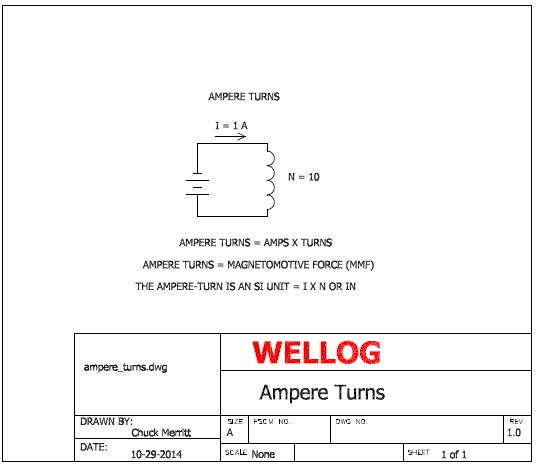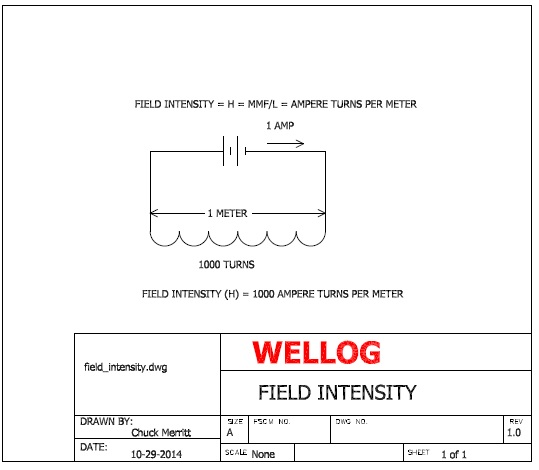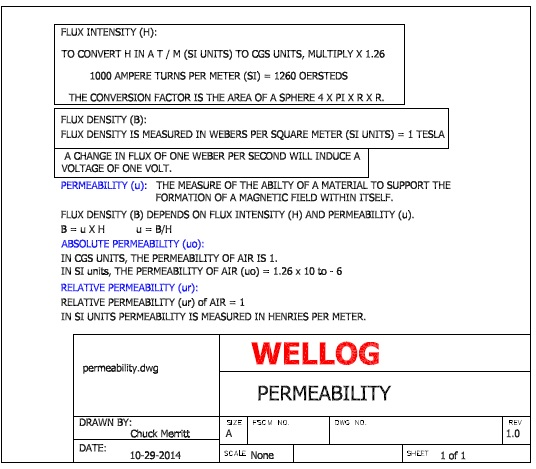WELLOG                                        ELECTROMAGNETISM

A basic understanding of electromagnetic theory is essential to appreciation of the capabilities and limitations of electromagnetism in geophysics.

BASIC ELECTROMAGNETIC THEORY:

The following illustration shows a simple circuit having a power source connected to an inductor having a number of turns (N).

In this case, the number of turns is 10. The Source of power is a battery and given a certain circuit resistance, the current (I) through the inductor is 1 ampere.

It can also be appreciated that the current flow through the inductor creates a magnetic field. The magnetic field has a force known as Magnetomotive Force (MMF).

Further, the magnetomotive force is directly proportional to the current (I) and the Number of turns (N) such that MMF = N x I.Induction coils used in Geophysical applications provide magnetic energy intensity referred to in terms of a magnetic “Moment”.

As shown in the following illustration, the Moment is the product of Number of turns (N), Current (I), and Area (A) in meters.

The area (A) is a measurement of the area of the loop. In this example, a loop having an area of 1 meter, 1000 turns and current of 1 ampere

Imposes a magnetic Moment (N x I x A) of 1000 Newtons.Additional considerations include Magnetic intensity in CGS units and the conversion

As shown in the following illustration.SUMMARY:

The capability of an electromagnetic system in geophysics is directly dependent on the magnetomotive force and area of the coil system employed.

APPLICATIONS:

Electromagnetism is applied in many geophysical methods. The principals of electromagnetic induction are applied in airborne, surface and borehole logging systems.

Electromagnetic methods include a number of surface geophysical applications. Surface geophysical applications include Frequency Domain systems and Time Domain Systems.

FREQUENCY DOMAIN:

Frequency Domain systems use continuous wave, usually sine wave transmitters. The receiver usually is designed to measure in-phase (I) and quadrature (Q) portions of the signal.

TIME DOMAIN:

Time Domain systems usually transmit an electromagnetic pulse. The pulse enters the surrounding ground and creates eddy currents in conductors. The Eddy currents in the conductors generate a secondary magnetic field that is measured by receiver electronics.

WELLOG has webpages on this website that are directed toward specific applications related to electromagnetic methods.

Contact WELLOG at info@wellog.com with your questions on the use of EM methods in Geophysics.